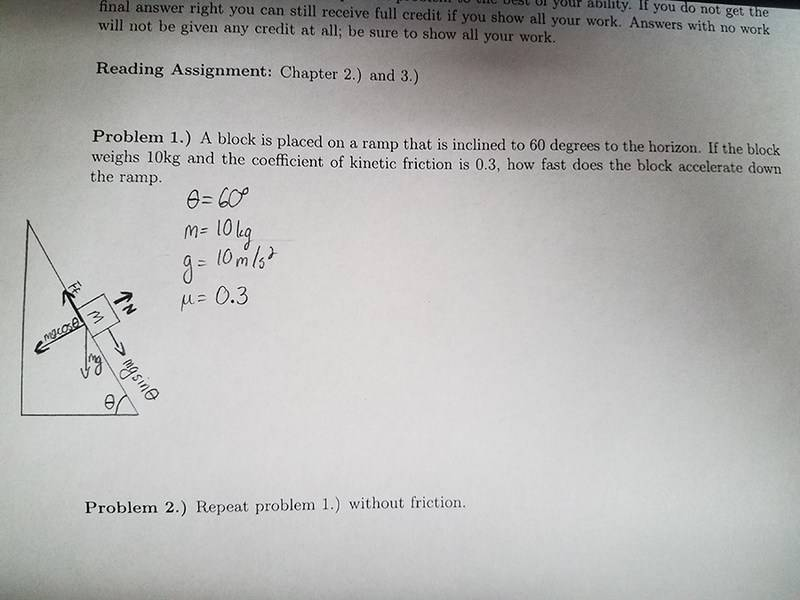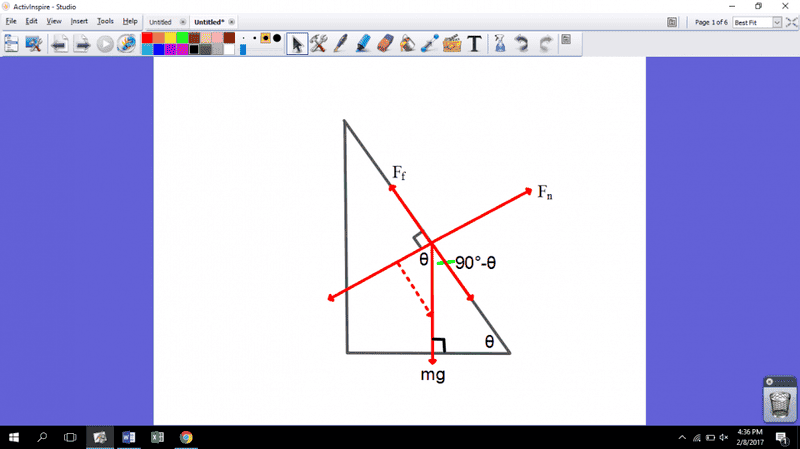# Why is this force the mgCos while the other is mgSin?

## Homework Statement

A block is placed on a ramp that is inclined to 60 degrees to the horizon. If the block weighs 10kg and the coefficient of kinetic friction is 0.3, how fast does the block accerlate down the ramp?

Theta=60 degrees
m=10kg
g=10m/s^2
mu=0.3

## The Attempt at a Solution

So far, all I have is the diagram that he gave us in class. I can somewhat tell why we're using the trig functions, but what I'm curious about is why mgsin(theta) is the block sliding down the ramp and mgcos(theta) is the reactionary force of gravity. Why not the other way around?QuantumQuest
Gold Member
Draw the vertical lines from the down edge of mg to the opposite sides of plane and see which angle is ##\theta## in the right triangle that is formed. In this triangle, find each vertical side using trigonometry.This is where theta is to be placed. If you understand why, try to figure it out from there.

CWatters